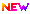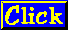# SSC GD Number and Letter Series 100 Bits

## SSC GD Number and Letter Series 100 Bits

1) QPO, NML, KJI, _____, EDC

A. HGF
B. CAB
C. JKL
D. GHI

Explanation:

This series consists of letters in a reverse alphabetical order.

2) JAK, KBL, LCM, MDN, _____

A. OEP
B. NEO
C. MEN
D. PFQ

Explanation:

This is an alternating series in alphabetical order. The middle letters follow the order ABCDE. The first and third letters are alphabetical beginning with J. The third letter is repeated as a first letter in each subsequent three-letter segment.

3) BCB, DED, FGF, HIH, ___

A. JKJ
B. HJH
C. IJI
D. JHJ

Explanation:

This series consists of a simple alphabetical order with the first two letters of all segments: B, C, D, E, F, G, H, I, J, K. The third letter of each segment is a repetition of the first letter.

4) QAR, RAS, SAT, TAU, _____

A. UAV
B. UAT
C. TAS
D. TAT

Explanation:

In this series, the third letter is repeated as the first letter of the next segment. The middle letter, A, remains static. The third letters are in alphabetical order, beginning with R.

5) SCD, TEF, UGH, ____, WKL

A. CMN
B. UJI
C. VIJ
D. IJT

Explanation:

There are two alphabetical series here. The first series is with the first letters only: STUVW. The second series involves the remaining letters: CD, EF, GH, IJ, KL.

6) FAG, GAF, HAI, IAH, ____

A. JAK
B. HAL
C. HAK
D. JAI

Explanation:

The middle letters are static, so concentrate on the first and third letters. The series involves an alphabetical order with a reversal of the letters. The first letters are in alphabetical order: F, G, H, I , J. The second and fourth segments are reversals of the first and third segments. The missing segment begins with a new letter.

7) ELFA, GLHA, ILJA, _____, MLNA

A. OLPA
B. KLMA
C. LLMA
D. KLLA

Explanation:

The second and forth letters in the series, L and A, are static. The first and third letters consist of an alphabetical order beginning with the letter E.

8) CMM, EOO, GQQ, _____, KUU

A. GRR
B. GSS
C. ISS
D. ITT

Explanation:

The first letters are in alphabetical order with a letter skipped in between each segment: C, E, G, I, K. The second and third letters are repeated; they are also in order with a skipped letter: M, O, Q, S, U.

9) Look at this series: 7, 10, 8, 11, 9, 12, … What number should come next?

A. 7
B. 10
C. 12
D. 13

Explanation:

This is a simple alternating addition and subtraction series. In the first pattern, 3 is added; in the second, 2 is

10) Look at this series: 36, 34, 30, 28, 24, … What number should come next?

A. 20
B. 22
C. 23
D. 26

Explanation:

This is an alternating number subtraction series. First, 2 is subtracted, then 4, then 2, and so on.

11) Look at this series: 22, 21, 23, 22, 24, 23, … What number should come next?

A. 22
B. 24
C. 25
D. 26

Explanation:

In this simple alternating subtraction and addition series; 1 is subtracted, then 2 is added, and so on.

12) Look at this series: 53, 53, 40, 40, 27, 27, … What number should come next?

A. 12
B. 14
C. 27
D. 53

Explanation:

In this series, each number is repeated, then 13 is subtracted to arrive at the next number.

13) Look at this series: 21, 9, 21, 11, 21, 13, 21, … What number should come next?

A. 14
B. 15
C. 21
D. 23

Explanation:

In this alternating repetition series, the random number 21 is interpolated every other number into an otherwise simple addition series that increases by 2, beginning with the number 9.

14) Look at this series: 58, 52, 46, 40, 34, … What number should come next?

A. 26
B. 28
C. 30
D. 32

Explanation:

This is a simple subtraction series. Each number is 6 less than the previous number.

15) Look at this series: 3, 4, 7, 8, 11, 12, … What number should come next?

A. 7
B. 10
C. 14
D. 15

Explanation:

This alternating addition series begins with 3; then 1 is added to give 4; then 3 is added to give 7; then 1 is added,

16) Look at this series: 8, 22, 8, 28, 8, … What number should come next?

A. 9
B. 29
C. 32
D. 34

Explanation:

This is a simple addition series with a random number, 8, interpolated as every other number. In the series, 6 is added to each number except 8, to arrive at the next number.SSC GD General Knowledge Questions and Answers -1SSC GD General Knowledge Questions and Answers-2SSC GD English Previous Questions Part-1SSC GD English Previous Questions Part-2

17) Look at this series: 14, 28, 20, 40, 32, 64, … What number should come next?

A. 52
B. 56
C. 96
D. 128

Explanation:

This is an alternating multiplication and subtracting series: First, multiply by 2 and then subtract 8.

18) Look at this series: 201, 202, 204, 207, … What number should come next?

A. 205
B. 208
C. 210
D. 211

Explanation:

In this addition series, 1 is added to the first number; 2 is added to the second number; 3 is added to the third number; 4 is added to the fourth number; and go on.

19) Look at this series: 544, 509, 474, 439, … What number should come next?

A. 404
B. 414
C. 420
D. 445

Explanation:

20) This is a simple subtraction series. Each number is 35 less than the previous number.

Look at this series: 80, 10, 70, 15, 60, … What number should come next?

A. 20
B. 25
C. 30
D. 50Top 70 General Science Questions and AnswersDefence General science Important QuestionsSSC GD Constable General Awareness Questions PDFSSC GD Constable General Awareness Questions PDF – 2

Explanation:

This is an alternating addition and subtraction series. In the first pattern, 10 is subtracted from each number to arrive at the next. In the second, 5 is added to each number to arrive at the next.

21) Look at this series: 2, 6, 18, 54, … What number should come next?

A. 108
B. 148
C. 162
D. 216

Explanation:

This is a simple multiplication series. Each number is 3 times more than the previous number.

22) Look at this series: 8, 6, 9, 23, 87 , … What number should come next?

A. 128
B. 226
C. 324
D. 429Download 100 Questions  and Answers PDF